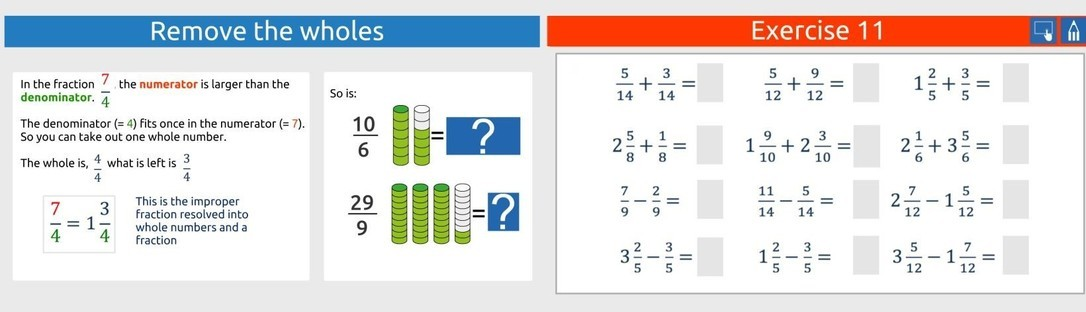Calculate with fractions part 2

View this page on a larger screen to send this lesson directly to Presenter

Purpose

• Learn about fractions
• What a fraction is and their parts.
• The concepts of numerator and denominator.
• What equivalent fractions are.
• How to organize fractions.
• How to simplify fractions.
• What improper fractions are.
• How to resolve an improper fraction.
• How to create fractions with the same name.
• Adding and subtracting similar and dissimilar fractions.
• Multiplying fractions.
• Dividing fractions.